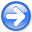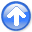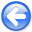Fast log-decon with a quasi-Newton solver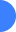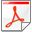Next: An efficient algorithm for Up: APPENDIX Previous: APPENDIX

## The limited-memory BFGS method

Nocedal (1980) derives a technique that partially solves the storage problem caused by the BFGS update. Instead of keeping all the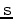and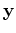from the past iterations, we update the Hessian using the information from theprevious iterations, whereis given by the end-user. This implies that when the number of iterations is smaller than, we have the usual BFGS update, and when it is larger than, we have a limited-memory BFGS (L-BFGS) update.

I give the updating formulas of the Hessian as presented by Nocedal (1980). First, we define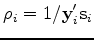,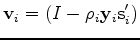andAs described above, when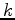, the iteration number, obeys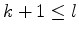, whereis the storage limit, we have the BFGS update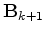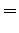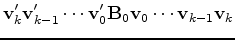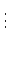(4)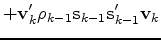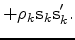Forwe have the limited-memory update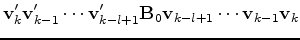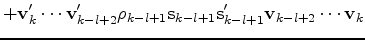(5)These equations show how the update of the Hessian is calculated.

Usually the L-BFGS method is implemented with a line search for the step length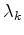to ensure a sufficient decrease of the misfit function. Convergence properties of the L-BFGS method are guaranteed ifin equation (2) satisfies the Wolfe conditions (Kelley, 1999):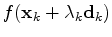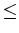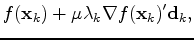(6)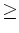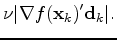(7)and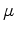are constants to be chosen a priori and. Forandwe set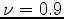and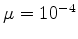as proposed by Liu and Nocedal (1989). Equation (6) is a sufficient decrease condition that all line search algorithms must satisfy. Equation (7) is a curvature condition. The line search algorithm has to be carefully designed since it absorbs most of the computing time. I programmed a line search based on the More and Thuente (1994) method. Because the line search is time consuming, the step length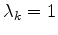is always tested first. This procedure saves a lot of computing time and is also recommended by Liu and Nocedal (1989). I now give the algorithm used to minimize any objective function involving nonlinear problems.Fast log-decon with a quasi-Newton solverNext: An efficient algorithm for Up: APPENDIX Previous: APPENDIX

2012-10-29